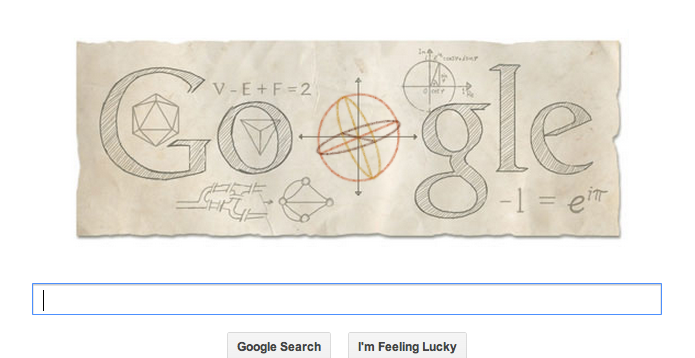## You're reading: Posts Tagged: leonhard euler

### Cushing your luck: properties of randomly chosen numbers

Long-time Aperiodical muse David Cushing has made a bet with us that he can give us an interesting post every Friday for the next ten weeks. Every week that he sends a post, we buy him a bar of chocolate. Every week that he doesn’t send us a post, he buys us a bar of chocolate. For his first trick, David is going to do some unnatural things with the natural numbers.

The greatest common divisor (gcd) of two or more integers is the greatest integer that evenly divides those integers. For example, the gcd of $8$ and $12$ is $4$ (usually written as $\gcd(8,12)=4$). Two integers are called coprime (or “relatively prime”) if their gcd is equal to $1$.

A reasonable question to ask is,

Given two randomly chosen integers $a$ and $b$, what is the probability that $\gcd(a,b)=1$?

### Happy Birthday Euler!Today is Euler’s $-306 \times e^{i \pi}$th birthday, and Google have chosen to celebrate (despite ignoring several other prominent mathematical birthdays, including Erdős’s centenary – see the @MathsHistory twitter feed for a full list) by creating a Google doodle on their homepage.

For anyone who isn’t aware, this is when Google changes the image above the search box on the homepage at Google.com, so it still says ‘Google’ but using an appropriate image, which sometimes has built-in interactive elements. I thought it was worth pointing out some of the fantastic maths they’ve included in today’s doodle.

### Open Season – The Perfect Cuboid

In this short series of articles, I’m writing about mathematical questions we don’t know the answer to – which haven’t yet been proven or disproven. This is the second article in the series, and considers a less well-known variant on an extremely well-known problem.

Ask anyone to name a theorem, and they’ll probably come up with one of the really famous ones, like Pythagoras’ theorem. This super-handy hypotenuse fact states that for a triangle with sides A, B and C, where the angle between A and B is a right angle, we have $C^2 = A^2 + B^2$. This leads us on to a nice bit of stamp-collecting – there are infinitely many triples of integers, A, B and C, which fit this equation, called Pythagorean Triples.

One well-known generalisation of this is to change the value $2$ to larger values, and go looking for triples satisfying $C^n = A^n + B^n$. But don’t – Andrew Wiles spent a good chunk of his life on proving that you can’t, for any value of $n>2$, find any such triples. The statement was originally made by Pierre De Fermat, and while Fermat famously didn’t write down a proof, it was the last of his mathematical statements to be gifted one – hence the name ‘Fermat’s Last Theorem’ – and proving it took over 350 years.